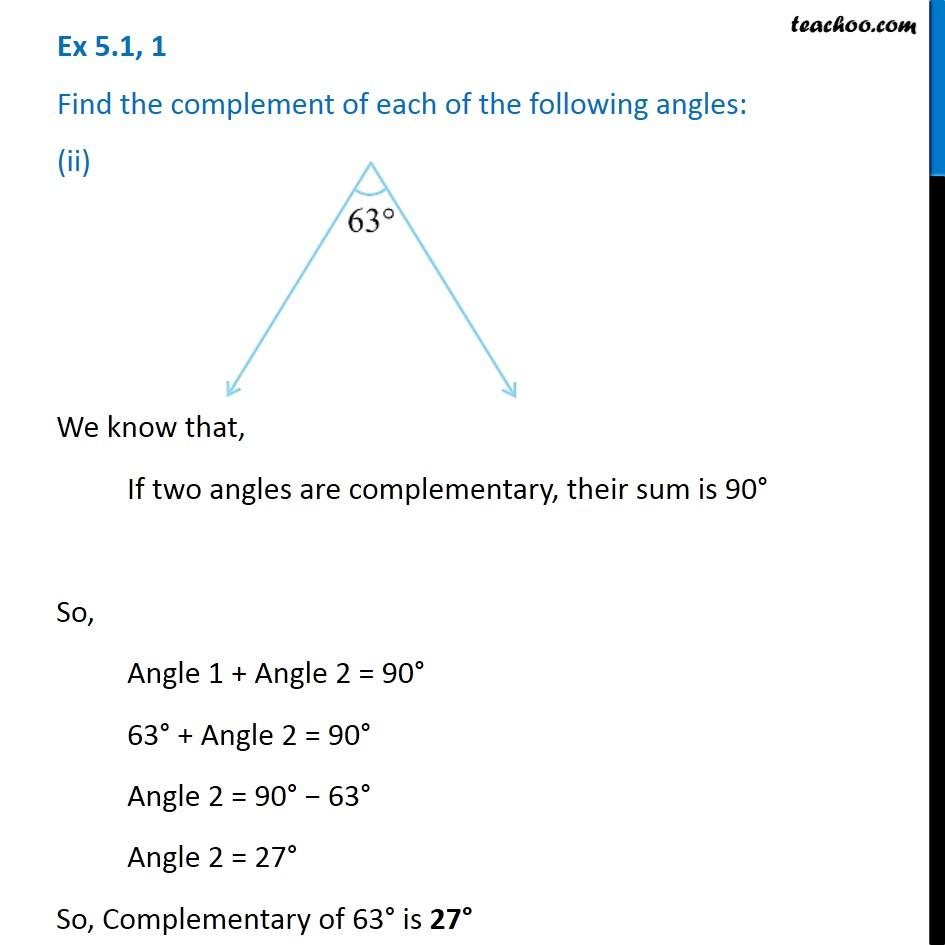Ex 5.1

Chapter 5 Class 7 Lines and Angles
Serial order wiseIntroducing your new favourite teacher - Teachoo Black, at only ₹83 per month

### Transcript

Ex 5.1, 1 Find the complement of each of the following angles: (ii) We know that, If two angles are complementary, their sum is 90° So, Angle 1 + Angle 2 = 90° 63° + Angle 2 = 90° Angle 2 = 90° − 63° Angle 2 = 27° So, Complementary of 63° is 27°